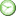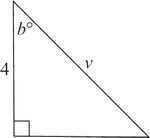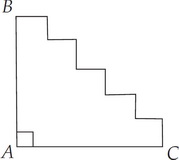# ISEE Math Practice Test: Upper Level Only

### Test Information15 questions0 minutes

Take more free ISEE Mathematics Achievement Practice Tests available from crackssat.com.

1. If b = 45, then v2 =• A. 32
• B. 25
• C. 16
• D. 5

2. One-half of the difference between the number of degrees in a square and the number of degrees in a triangle is

• A. 45
• B. 90
• C. 180
• D. 240

3. If the area of a square is equal to its perimeter, what is the length of one side?

• A. 1
• B. 2
• C. 4
• D. 8

4. The area of a rectangle with width 4 and length 3 is equal to the area of a triangle with a base of 6 and a height of

• A. 1
• B. 2
• C. 3
• D. 4

5. Two cardboard boxes have equal volume. The dimensions of one box are 3 × 4 × 10. If the length of the other box is 6 and the width is 4, what is the height of the second box?

• A. 2
• B. 5
• C. 10
• D. 12

6. If the area of a square is 64p2, what is the length of one side of the square?

• A. 64p2
• B. 8p2
• C. 64p
• D. 8p

7.If AB = 10 and AC = 15, what is the perimeter of the figure above?

• A. 25
• B. 35
• C. 40
• D. 50

8.If ABCD is a rectangle, what is the value of w + x + y + z ?

• A. 90
• B. 150
• C. 180
• D. 190

9.What is the area of the figure above if all the angles shown are right angles?

• A. 38
• B. 42
• C. 50
• D. 88

10. How many meters of police tape are needed to wrap around a rectangular crime scene that measures 6 meters wide by 28 meters long?

• A. 34 meters
• B. 68 meters
• C. 90 meters
• D. 168 meters

11.The distance between points A and B in the coordinate plane above is

• A. 5
• B. 6
• C. 8
• D. 10

12.PO and QO are radii of the circle with center O. What is the value of x ?

• A. 30
• B. 45
• C. 60
• D. 90

13.What is the value of x ?

• A. 360
• B. 100
• C. 97
• D. 67

14.ABC is an equilateral triangle. What is the perimeter of this figure?

• A. 4 + 2π
• B. 4 + 4π
• C. 8 + 2π
• D. 8 + 4π

15.In trapezoid LMNO, line LO has a length of 20. What is the area of the trapezoid?

• A. 48
• B. 64
• C. 88
• D. 112